• 西储大学轴承数据matlab
2022-05-07 21:27:11

### 一概述

##### 1.1说明

极限学习机的实现程序。
处理的数据为西储大学的不同故障类型的轴承数据。
程序包括：预处理数据和matlab训练预测部分
原理：参考博客

##### 1.2开发工具

matlab2018
pycharm2020
python版本：python3.7（重要）

### 二.程序实现

用python分段截取西储大学的原始数据，再用matlab提取为特征值，通过极限学习机训练和预测。
PS：程序中涉及的路径请改为自己的西储大学轴承数据文件的路径。

##### （1）数据预处理一（python）

请先下载数据
西储大学数据
PS：如果python运行有问题，请将python更新到3.7版本
python分段截取西储大学的原始数据

import numpy as np
import scipy.io as scio
from random import shuffle

def normalize(data):
'''(0,1)normalization
:param data : the object which is a 1*2048 vector to be normalized
'''
s= (data-min(data)) / (max(data)-min(data))

return  s

def cut_samples(org_signals):
''' get original signals to 10*120*2048 samples, meanwhile normalize these samples
:param org_signals :a 10* 121048 matrix of ten original signals
'''

results=np.zeros(shape=(10,120,2048))
temporary_s=np.zeros(shape=(120,2048))

for i in range(10):  #对应十类故障数据
s=org_signals[i]
for x in range(120):  #每类数据120段样本
temporary_s[x]=s[1000*x:2048+1000*x]  #每个样本信号长度1000
# temporary_s[x]=normalize(temporary_s[x])     #顺便对每个样本归一化
results[i]=temporary_s

return results

def make_datasets(org_samples):
'''输入10*120*2048的原始样本，输出带标签的训练集(占75%)和测试集(占25%)'''

train_x=np.zeros(shape=(10,90,2048))
train_y=np.zeros(shape=(10,90,10))
test_x=np.zeros(shape=(10,30,2048))
test_y=np.zeros(shape=(10,30,10))
for i in range(10):
s=org_samples[i]
#
index_s = [a for a in range(len(s))]
#shuffle(index_s) #打乱顺序
s=s[index_s]
# 对每种类型都划分训练集和测试集
train_x[i]=s[:90]
test_x[i]=s[90:120]
# 填写标签
label = np.zeros(shape=(10,))
label[i] = 1
train_y[i, :] = label
test_y[i, :] = label

#将十种类型的训练集和测试集分别合并并打乱
x1 = train_x
y1 = train_y
x2 = test_x
y2 = test_y
for i in range(9):
x1 = np.row_stack((x1, train_x[i + 1]))
x2 = np.row_stack((x2, test_x[i + 1]))
y1 = np.row_stack((y1, train_y[i + 1]))
y2 = np.row_stack((y2, test_y[i + 1]))

index_x1= [i for i in range(len(x1))]
index_x2= [i for i in range(len(x2))]
#shuffle(index_x1)
#shuffle(index_x2)
x1=x1[index_x1]
y1=y1[index_x1]
x2=x2[index_x2]
y2=y2[index_x2]

return x1, y1, x2, y2    #分别代表：训练集样本，训练集标签，测试集样本，测试集标签

# 读取原始数据，处理后保存
dataFile= 'H://deal_data1//c10signals.mat'
org_signals=data['signals']
org_samples=cut_samples(org_signals)
train_x, train_y, test_x, test_y=make_datasets(org_samples)

saveFile = 'H://deal_data1//datasets.mat'
scio.savemat(saveFile, {'train_x':train_x, 'train_y':train_y, 'test_x':test_x, 'test_y':test_y})


##### （2）数据预处理二（matlab）

将原始数据保存到一个文件

clc;
clear all;
close all;

drive_100 = load('H:\西储大学轴承数据中心网站\Normal Baseline Data\100.mat');
drive_108 = load('H:\西储大学轴承数据中心网站\12k Drive End Bearing Fault Data\108.mat');
drive_121 = load('H:\西储大学轴承数据中心网站\12k Drive End Bearing Fault Data\121.mat');
drive_133 = load('H:\西储大学轴承数据中心网站\12k Drive End Bearing Fault Data\133.mat');
drive_172 = load('H:\西储大学轴承数据中心网站\12k Drive End Bearing Fault Data\172.mat');
drive_188 = load('H:\西储大学轴承数据中心网站\12k Drive End Bearing Fault Data\188.mat');
drive_200 = load('H:\西储大学轴承数据中心网站\12k Drive End Bearing Fault Data\200.mat');
drive_212 = load('H:\西储大学轴承数据中心网站\12k Drive End Bearing Fault Data\212.mat');
drive_225 = load('H:\西储大学轴承数据中心网站\12k Drive End Bearing Fault Data\225.mat');
drive_237 = load('H:\西储大学轴承数据中心网站\12k Drive End Bearing Fault Data\237.mat');

% de_100 = drive_100.X100_DE_time(1:121048);
de_100 = drive_100.X100_DE_time(1:4:484192);
de_108 = drive_108.X108_DE_time(1:121048);
de_121 = drive_121.X121_DE_time(1:121048);
de_133 = drive_133.X133_DE_time(1:121048);
de_172 = drive_172.X172_DE_time(1:121048);
de_188 = drive_188.X188_DE_time(1:121048);
de_200 = drive_200.X200_DE_time(1:121048);
de_212 = drive_212.X212_DE_time(1:121048);
de_225 = drive_225.X225_DE_time(1:121048);
de_237 = drive_237.X237_DE_time(1:121048);

de_signals = [de_100,de_108,de_121,de_133,de_172,de_188,de_200,de_212,de_225,de_237];
signals = de_signals.';
save('H:\你的文件夹\c10signals.mat','signals');
whos('-file','H:\你的文件夹\deal_data1\c10signals.mat')

##### （3）提取特征（matlab）

matlab 提取轴承原始数据为特征值

load H:\研究生文件\极限学习机\所有数据\deal_data1\datasets.mat
%分类为1-10 十类故障信号
NumberOfFeature=5;

[m_trx,n_trx]=size(train_x);
[m_try,~]=size(train_y);
[m_tx,n_tx]=size(test_x);
[m_ty,~]=size(test_y);

%生成零矩阵提取特征零矩阵
ttrain_x=zeros(m_trx,NumberOfFeature);
ttrain_y=zeros(m_try,1);
ttest_x=zeros(m_tx,NumberOfFeature);
ttest_y=zeros(m_ty,1);

%把训练数据分类保存为n行1列数据（由于原始数据的格式是n行10列）
for i=1:m_try
col=find(train_y(i,:)~=0);
ttrain_y(i,1)=col;
end
for i=1:m_ty
col=find(test_y(i,:)~=0);
ttest_y(i,1)=col;
end

%均值 第一列
ttrain_x(:,1)=mean(train_x,2);%按行平均
ttest_x(:,1)=mean(test_x,2);%按行平均
%方差 第二列
ttrain_x(:,2)=std(train_x,0,2);
ttest_x(:,2)=std(test_x,0,2);
%最大值
ttrain_x(:,3)=max(train_x,[],2);
ttest_x(:,3)=max(test_x,[],2);
%最小值 第四列
ttrain_x(:,4)=min(train_x,[],2);
ttest_x(:,4)=min(test_x,[],2);

for i=1:m_trx
frequencystruct  = frequencyDomainFeatures(train_x(i,:)',12000);
ttrain_x(i,5)=frequencystruct.MF';%平均频率
% ttrain_x(i,6)=frequencystruct.FC';%重心频率
% ttrain_x(i,7)=frequencystruct.RMSF'; %频率均方根
% ttrain_x(i,8)=frequencystruct.RVF'; %频率标准差
end
for i=1:m_tx
frequencystruct  = frequencyDomainFeatures(test_x(i,:)',12000);
ttest_x(i,5)=frequencystruct.MF';%平均频率
%ttest_x(i,6)=frequencystruct.FC';%重心频率
% ttest_x(i,7)=frequencystruct.RMSF'; %频率均方根
% ttest_x(i,8)=frequencystruct.RVF'; %频率标准差
end
%归一化
% ttrain_x=mapminmax(ttrain_x,-1,1);
% ttest_x=mapminmax(ttest_x,-1,1);

ttrain_x=roundn(ttrain_x,-4);
ttrain_y=roundn(ttrain_y,-4);
ttest_x=roundn(ttest_x,-4);
ttest_y=roundn(ttest_y,-4);

save('H:\研究生文件\极限学习机\所有数据\deal_data1\zhouc_data.mat','ttrain_x','ttrain_y','ttest_x','ttest_y');
xlswrite('H:\deal_data1\ttrain_x.xlsx',ttrain_x);
xlswrite('H:\deal_data1\ttrain_y.xlsx',ttrain_y);
xlswrite('H:\deal_data1\ttest_x.xlsx',ttest_x);
xlswrite('H:\deal_data1\ttest_y.xlsx',ttest_y);


##### （4）极限学习机训练与预测

主程序：

%% I. 清空环境变量
clear all
clc
%%分类数据有10类就是0-9
%% II. 训练集/测试集产生
%%
% 1. 导入数据
%
%%
% 2. 随机选择数据作为训练集和测试集

P_train = ttrain_x';
T_train = ttrain_y';
P_test = ttest_x';
T_test = ttest_y';

%% III. ELM创建/训练
[IW,B,LW,TF,TYPE] = elmtrain(P_train,T_train,30,'sig',1);

%% IV. ELM仿真测试
T_sim_1 = elmpredict(P_train,IW,B,LW,TF,TYPE);%结果是行矩阵 1*120矩阵
T_sim_2 = elmpredict(P_test,IW,B,LW,TF,TYPE);

%% V. 结果对比
result_1 = [T_train' T_sim_1'];%
result_2 = [T_test' T_sim_2'];
%%
% 1. 训练集正确率
k1 = length(find(T_train == T_sim_1));
n1 = length(T_train);
Accuracy_1 = k1 / n1 * 100;
disp(['训练集正确率Accuracy = ' num2str(Accuracy_1) '%(' num2str(k1) '/' num2str(n1) ')'])

%%
% 2. 测试集正确率
k2 = length(find(T_test == T_sim_2));
n2 = length(T_test);
Accuracy_2 = k2 / n2 * 100;
disp(['测试集正确率Accuracy = ' num2str(Accuracy_2) '%(' num2str(k2) '/' num2str(n2) ')'])

%% VI. 绘图
figure(2)
plot(1:300,T_test,'bo',1:300,T_sim_2,'r-*')
grid on
xlabel('测试集样本编号')
ylabel('测试集样本类别')
string = {'测试集预测结果对比(ELM)';['(正确率Accuracy = ' num2str(Accuracy_2) '%)' ]};
title(string)
legend('真实值','ELM预测值')


elmtrain.m函数

function [IW,B,LW,TF,TYPE] = elmtrain(P,T,N,TF,TYPE)
%输出是IW B LW
% ELM训练
% [IW,B,LW,TF,TYPE] = elmtrain(Pn_train,Tn_train,30,'sig',0);
% Description
% Input
% P   - Input Matrix of Training Set  (R*Q)  %属性矩阵 4*120
% T   - Output Matrix of Training Set (S*Q)  %类别矩阵 1*120
% N   - Number of Hidden Neurons (default = Q) %隐藏层神经元数据
% TF  - Transfer Function:
%       'sig' for Sigmoidal function (default)
%       'sin' for Sine function
%       'hardlim' for Hardlim function
% TYPE - Regression (0,default) or Classification (1)
% Output
% IW  - Input Weight Matrix (N*R)
if nargin < 2 %nargin 参数数量
error('ELM:Arguments','Not enough input arguments.');
end
if nargin <3
N = size(P,2);
end

if nargin <4
TF = 'sig';
end

if nargin < 5
TYPE = 0;
end

if size(P,2) ~= size(T,2)
error('ELM:Arguments','The columns of P and T must be same.');
end

[R,Q] = size(P);
if TYPE  == 1
T  = ind2vec(T+ones(size(T)));%首先从字面上的意思ind2vec()函数表示从索引到向量 ones(size(T)原来是没有的
%原来的T是150*1矩阵，新的T变成3*150矩阵
%disp('TYPE -in你好！');
end

[S,Q] = size(T);

%随机产生输入层权重矩阵
IW = rand(N,R) * 2 -1 ; %20*4
%随机产生隐含层偏置矩阵
B = rand(N,1);
BiasMatrix = repmat(B,1,Q); %重复数组 这里的repmat(B,1,Q); 将矩阵B重复到 1×Q 块排列中。相当于重排列列为N*Q 即20*120
%计算隐含层权值矩阵
tempH = IW*P+BiasMatrix; %IW是20*4 P是4*120 IW*P是20*120矩阵 BiasMatrix是
switch TF
case 'sig' %采用sig函数  激活函数
H = 1 ./ (1 + exp(-tempH));
case 'sin'
H = sin(tempH);
case 'hardlim'
H = hardlim(tempH);
end

% Calculate the Output Weight Matrix

LW = pinv(H') * T'; %pinv求伪逆矩阵  这里的是转置矩阵，因为实数情况下，共轭转置和转置没区别

end



elmpredict.m函数

function Y = elmpredict(P,IW,B,LW,TF,TYPE)
% ELMPREDICT Simulate a Extreme Learning Machine
% Syntax
% Y = elmtrain(P,IW,B,LW,TF,TYPE)
% Description
% Input
% P   - Input Matrix of Training Set  (R*Q)
% IW  - Input Weight Matrix (N*R)
% B   - Bias Matrix  (N*1)
% LW  - Layer Weight Matrix (N*S)
% TF  - Transfer Function:
%       'sig' for Sigmoidal function (default)
%       'sin' for Sine function
%       'hardlim' for Hardlim function
% TYPE - Regression (0,default) or Classification (1)
if nargin < 6
error('ELM:Arguments','Not enough input arguments.');
end
% Calculate the Layer Output Matrix H
Q = size(P,2);
BiasMatrix = repmat(B,1,Q);
tempH = IW * P + BiasMatrix;

switch TF
case 'sig'
H = 1./ (1+exp(-tempH));

case 'sin'
H = sin(tempH);

case 'hardlim'
H = hardlim(tempH);
end
%计算模拟输出
Y = (H' * LW)';
if TYPE == 1 %执行
temp_Y = zeros(size(Y));
for i = 1:size(Y,2) %Y的列数
[~,index] = max(Y(:,i)); %第i列 index获得最大值的位置 ~是最大值
temp_Y(index,i) = 1;
end
Y = vec2ind(temp_Y); %向量转索引
end

Y=Y;matlab python 机器学习
更多相关内容
• 包含了西储大学官网上下载的数据两个版本，方便需要搜集数据的科研人员；2.包含了1个说明word，可以知道每一个文件的实际工况，比如是轴承内圈或外圈故障或滚子故障；3.包含了原创设计的GUI界面，方便做进一步分析。...
• 包含的资料有：西储大学轴承数据+中英文版本的数据说明+matlab加载和处理轴承数据的案例和方法+利用EMD、Hilbert和FFT处理轴承数据信号的程序，并且有解释； 同时还附带了EMD、ITD、VMD等算法的程序，其中EMD程序有...
• 针对西储大学轴承故障数据进行了EMD分解，并对故障特征频率等信息进行了提取
• 小波降噪，运用小波工具将图像进行分层处理，可以得到降噪后的图像，含有凯斯西储大学轴承数据
• 本资源整理了西储大学轴承的各项数据以及数据说明，内容清晰全面，有助于各类需要人士进行分析研究，提高自己的科研水平。
• 整理好的西储大学轴承数据，包含各类工况和各类故障，内含图片和txt文本说明，希望能够给到各位帮助，后续会接着更新数据处理和深度学习代码部分。
• 网上有关于轴承故障特征频率计算的资源，但是东一句，西一句，比较零散，而且表述上不太明确，容易让初学者走弯路，特整理有关资料并上传，希望能帮助到大家！共同进步！
• 美国西储大学共享了其滚动轴承实验数据，但是利用MATLAB导入不方便，此函数是用于提高MATLAB导入数据的效率，使用者稍作修改即可使用。
•mat
• 轴承数据处理——1 参考公众号《故障诊断》

采用jupyter作为工具，python具体代码分析过程如下：首先取西储大学各类轴承数据如下：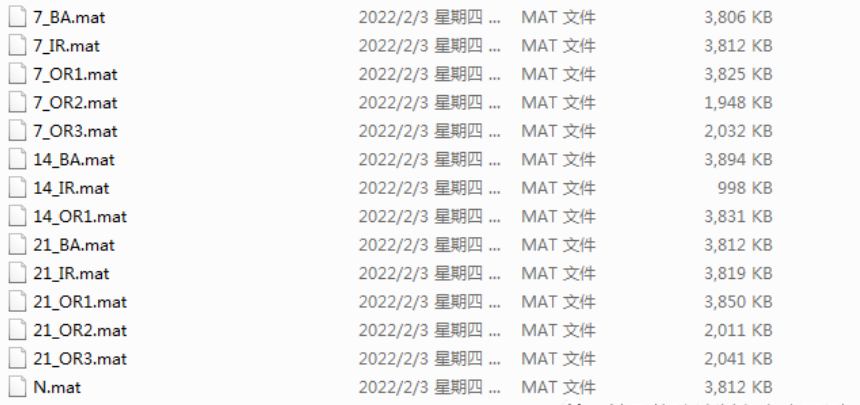导入所需的库函数：

import scipy.io # To use the '.mat' files
import seaborn as sns
import numpy as np
import pandas as pd
import os
import matplotlib.pyplot as plt

读取文件夹下所有数据：

for root, dirs, files in os.walk("0hp_load_48_KHz", topdown=False):
for file_name in files:
path = os.path.join(root, file_name)
print(path)

显示结果如下：

展开全文python
• 主要对滚珠轴承的状况分为四类：健康、滚动元件故障、内滚道故障、外滚道故障。 ，数据文件均为Matlab格式（.mat格式）。
• 西储大学轴承故障数据读取GUI,西储大学轴承数据说明,matlab源码.zip
• 西储大学轴承故障数据读取GUI,西储大学轴承数据说明,matlab源码.rar
• CWRU数据数据整理程序+下载好的数据包（防止程序中的下载部分出现bug）+数据使用说明，个人研究正在使用，可直接用（请将解压后的所有文件和故障诊断程序放在同一个目录下）
• 西储大学数据集介绍

## 0.写在前面的话

去年这个时候开始萌生了用深度学习进行机械故障诊断的想法，虽然目前勉强入门，但是回过头来看，确实走了不少弯路。整个学习过程几乎全靠网络上的资源，因此，写这篇文章除了回顾学习过程、总结提高，更重要的是也想帮到曾经像我一样迷茫的人。

## 1.针对的人群

说实话，当初我个人的起点真的是相当低，总想着找一些0基础入门的教程，无奈很多基础常识都很缺乏，学起来还是很吃力的。朋友们可以看一看我的条件，如果正准备开始入坑，相比之下应该能建立一些信心。

• 本科电子类，非机械，编程基础几乎为零

• 毕业十年后来脱产读研

• 导师非工科专业、第一次带研究生、平时很忙，更我无法指导我（一开始甚至不知道我的专业毕业要求。。。。）

• 没有师兄师姐！！！没有实验室！！！

• 已成家、诸事繁杂

• 天临加持，论文倒查十年，无法摸鱼

今年3月份开始总算是水了个EI会议、发了2个容易点的中文核心期刊，毕业算是稳一些了，整体效率还算可以。

所以打算往这个方向做研究的同志们完全不需要有心理负担，估计也没几个人比我读研的困难大了。

## 2.数据集介绍

### （1）西储大学数据集的优点

• 最大的优势就是用的人多，这样资源非常好找，也有很多人愿意分享自己的经验，论文多也好学习和比较。

• 数据集大小合适，只有650M，且包含各种工况、故障。（相比之下，好多数据集是轴承运行全程的数据，故障种类不全或者重叠，更适合做预测。如辛辛那提轴承数据、西安交通大学轴承数据等）

• 数据质量合适，能够有效区分出模型性能高低。（有些模型随便一整就是100%准确率，这就没法做文章了）

### （2）了解数据集

找到数据集比较简单，它长这个样子。数据集解压后看到的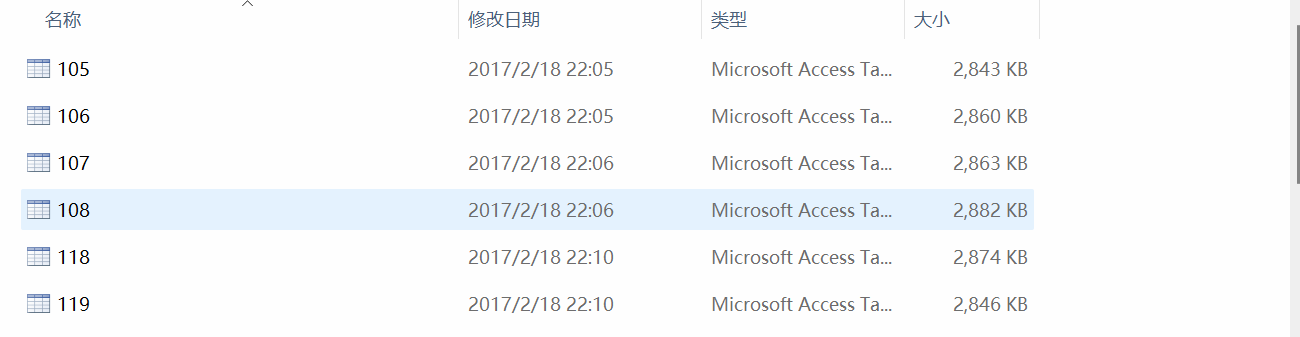12kHz DE端数据

我见过已经认为合并处理过的，但是不好找，个人觉得也没有必要，处理数据是必须要经历的一个学习过程。

首先它由4个文件夹组成，前3个分别是：12kHz采样率下电机端轴承的故障数据（DE）60个、12kHz采样率下风扇端轴承的故障数据（FE）45个、48kHz采样率下电机端轴承的故障数据（DE）52个，还有正常运转的轴承数据4个。以12kDE数据为例，大家基本也都在这个数据集下做验证。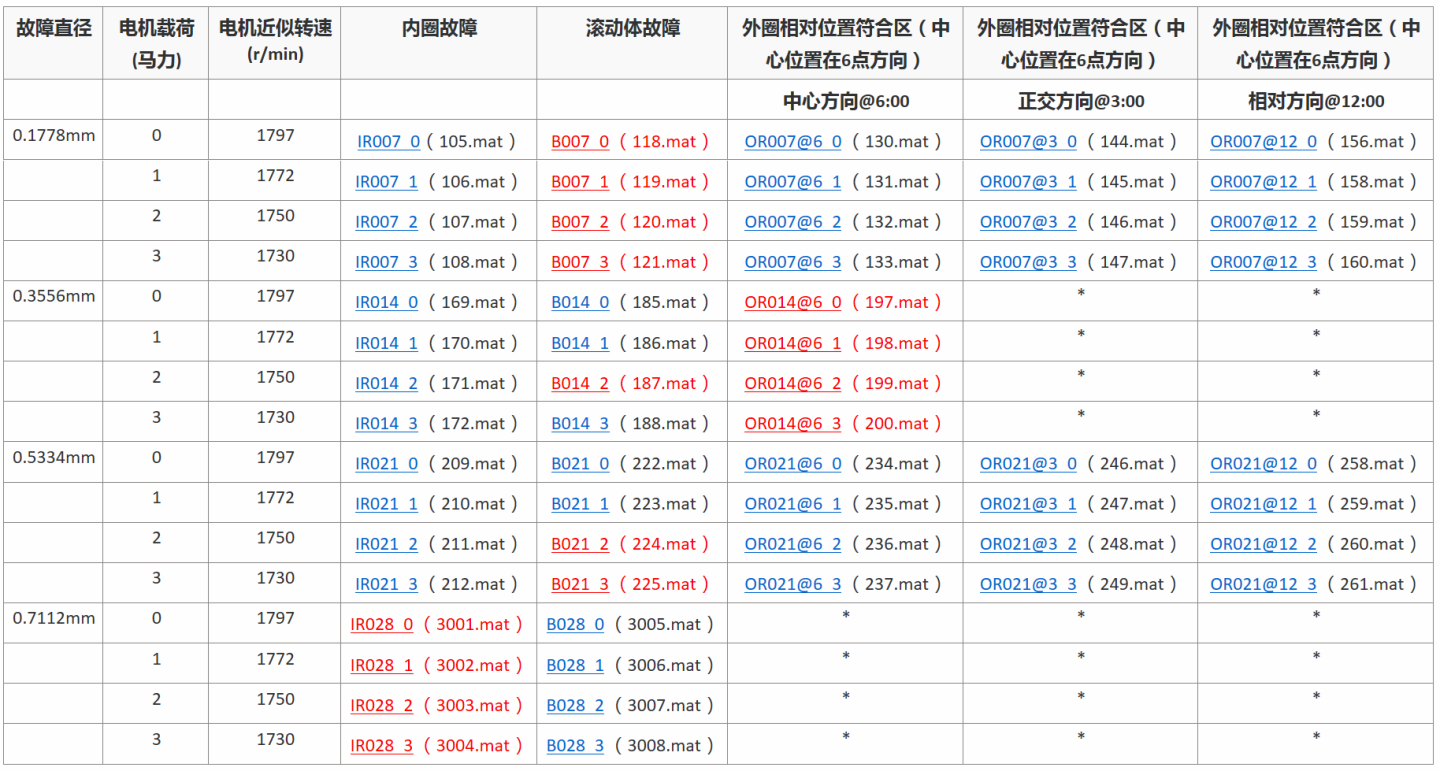​12kDE数据

如图所示，左边第一列是4种故障尺寸，由于第4种故障缺少外圈故障，所以一般选取前3种尺寸，这样内圈、滚动体、外圈3种故障就有3*3也就是9种故障分类，再加上正常状态就是最常见的10分类方式。不考虑故障尺寸进行4分类的比较少见，主要是这样的话难度降低不少，结果提升的空间不大，毕竟10分类也没有麻烦多少。（图中标红的请忽略，这几个数据在一篇论文中被认为是质量不佳甚至有问题的，有待进一步研究）

左边第二、第三列代表的就是常说的工况了，实际上就是负载、转速的不同，这就造成了数据一定程度的差异，这个差异不大，正好可以研究。区分工况是常见的做法，很多人取3种工况，也有取4种的，大家可以自己决定。我个人见的3种的多，主要用来做迁移学习或验证泛化能力，3种工况一般已经足够说明问题了。

外圈故障又细分为3种，建议大家取6点钟的就足够了，不同方向的差异时间上并不大，进行区分也没有那么多必要性。我在一开始为了包含所有情况，导致写的程序过于麻烦，浪费了不少时间，最后看来完全没有必要。

以上是12kDE数据，单独使用FE数据的我没见过，使用48kDE数据的也有，但确实不多，也没有必要，有论文研究指出这两组数据除了采样率不同，其余是几乎一样。

### （3）处理数据集

我之前没啥编程基础，所以准备直接用Python完成数据处理和模型搭建。当时这方面愿意分享Python程序的实在不多，很多是以前用Matlab语言，而我的Python基础不够，一开始就盯着几个博主写的模仿，实在是吃力。

首先文件的后缀是mat，用Python就能直接读取，可当你打开时才发现是三组数据？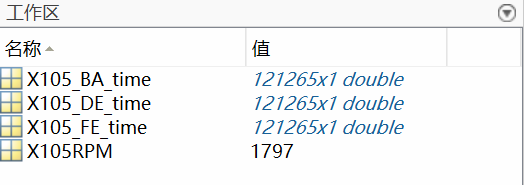matlab读取界面

在Python里会变成一组字典，可以根据名称选取需要的部分。

这里我有个疑问一直没看到确切说法，105.mat这个文件照理说是在12kDE里的，但是文件却同时包含了FE和BA保持架的数据，也就是说还得再进行一次选取，这里的FE数据不知道和12kFE文件里的是不是一回事。而且当你打开12kFE里的文件时，发现还是包含DE、FE和BA三种。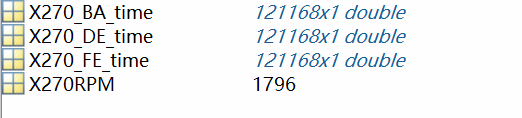​12kFE中的文件270.mat

打开正常数据是，发现是DE和FE两种。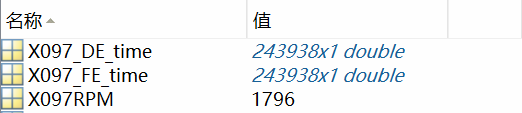正常运行的数据

目前就写到这里，后续再细说就要涉及具体的python程序了，比较麻烦，我得好好整理一下，希望大家多多支持，点赞加收藏。

展开全文• 凯斯西储大学轴承数据集.mat文件， 官网没有提供合集下载形式，是以表格形式呈现，手动下载需要分别建立文件夹，单独下载并改名，本资源已经将上述工作完成整合出压缩包供大家下载。
• 西储大学数据的处理其实不复杂，在网上用心搜索的话可以找到几个版本，但是在实际使用过程中多少都还会遇到一些问题。事实上，实现数据集的基础功能比较容易，但是如果在使用过程中有一些细节注意不到的话，很可能你...

西储大学数据的处理其实不复杂，在网上用心搜索的话可以找到几个版本，但是在实际使用过程中多少都还会遇到一些问题。事实上，实现数据集的基础功能比较容易，但是如果在使用过程中有一些细节注意不到的话，很可能你后续的实验会白做，这里我也走过一些弯路。大致来说，数据集制作一步到位比较难，因为后续你可能要在多种情况下应用，比如说加噪声、做FFT、维度变换、小波变换、选小样本进行迁移学习等。如果数据集搞不好，可能你的结果就不受控，到时候排查起问题来特别麻烦。

对于数据集的处理，我这里把它分为3个部分详细进行说明。

• 数据的读取

• 数据的分割组合

• 数据的变换

## 一、数据的读取

数据的读取是第一步，虽然文件的格式是mat，但是用matlab处理其实没有必要。我们直接用python自带的读取函数就可以，以最常见的10分类为例，即取工况1中，1个正常文件，3种尺寸的3种故障。这里很多人直接把1类文件放到一个文件夹里，我的则是分的更细些，把不同分类也用文件夹分开，如图所示。三种工况10种分类（也可以不分文件夹）

def readmat(path, label,name="_DE_time",steps=128):
datalist = []
mat_filename_list = os.listdir(path) #得到文件夹下的所有文件名称
mat_filename_list.sort(key=lambda x: int(x[:-4])) #文件名排序
for mat_filename in mat_filename_list:
matdata = scio.loadmat(path + mat_filename) #读取文件
data_name = "X" + mat_filename[0:3] + name  #提取所需的传感器数据
data = matdata[data_name] #提取所需的传感器数据
datalist.append(data)   #将数据整合至列表

我这里的代码是为了说明问题，并不完整，大家最好还是理解以后自行进行补全。

其实读取文件形成列表这一步大家都差不多，我这里在函数里增加了选项，可选DE或FE，可以用于后续做迁移学习。steps后续用来滑窗取值的参数，这里请大家先行自动忽略。

## 二、数据的分割组合

数据的分割组合是最重要的一个环节，在数据的划分比例上，也很难有统一的标准。这里一共有几个问题必须事先搞清楚。

1. 取多大的窗口？

2. 划分训练集、测试集还是训练集、验证集、测试集？

3. 各个数据集合的比例怎么定？

4. 要不要做滑窗取值？

首先我贴一张图，图里是我统计的个人看过的论文里的数据集划分方法，仅供参考。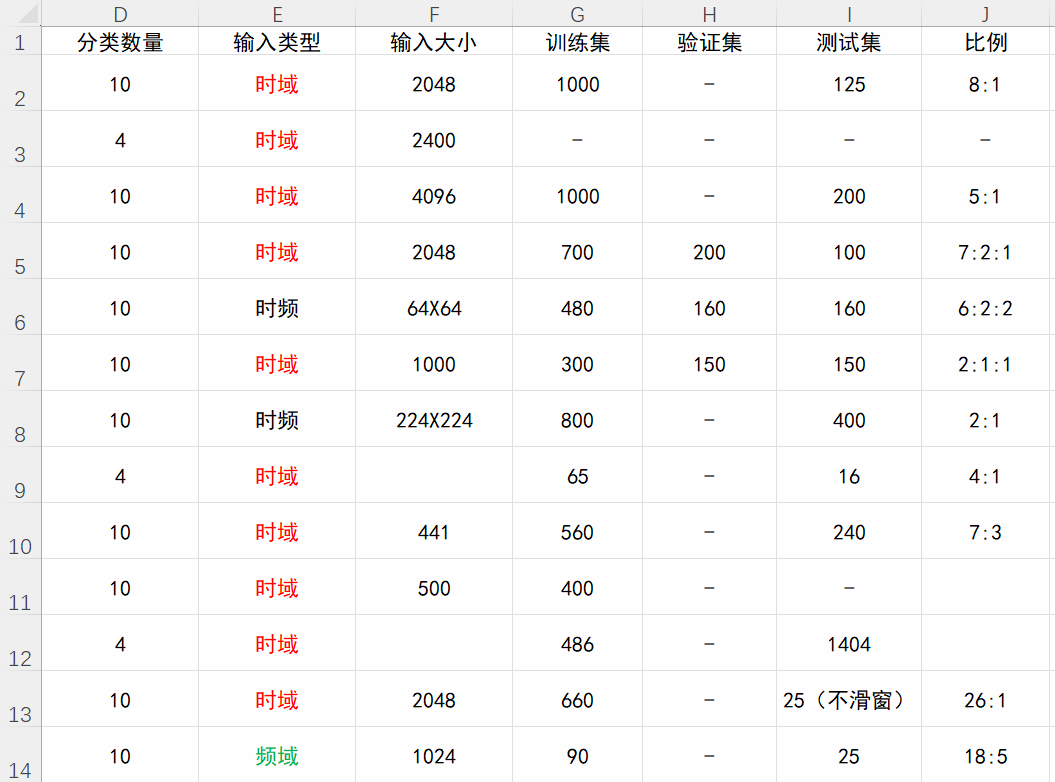数据集划分参考

上面的数据来源至少都是近几年中文核心，也有SCI、书籍、博士的研究，应该还算是比较有参考意义的。但是我们不难发现，大家虽然用的是同一个数据集，但划分方法咋就这么不一样呢？

1. 首先从分类讲，建议大家直接用10分类，因为4分类的指标普遍都太好了，做的人确实也较少，实际操作起来也不会简单多少，所以真的没这个必要。更何况从工程意义上讲，区分故障种类的同时我们肯定更希望知道故障的大致严重程度。

2. 输入数据的类型上就见仁见智了，令我没想到的是居然时域的分析比较多。时域分析的好处就是处理起来简单一些，缺点就是效果差一些（我自己测的），对噪声的鲁棒性差一些（也是我自己测的），大家可以自己试试。频域数据作为输入的在论文里我还没看到，这里统计的是在一本教材里看到的，作者是这个领域的博士，我觉得挺靠谱，自己也就借鉴了他的方法。频域数据的好处就是提取了频域信息，特征明显。毕竟是研究信号嘛，还是频域图更加直观，传统的方法里故障频率可是至关重要的指标。确定是丢失了时间特征，目前我感觉时间特征其实不是很关键，因为训练前大家都要随机排列样本的，而每一小段样本里的时间信息确实太少了，恐怕也难以说明问题；时频图作为输入的最近几年不少，就是通过小波变换或者短时傅里叶变换形成时频图，将图片作为输入。好处就是既保留了时域信息，也提取了频域特征。而图片的输入形式，天然适配各种成熟的图片处理网络模型，做起实验来省点事。缺点就是尺寸有点大，对我来说自己的笔记本电脑肯定跑不动，效果好不好我也没试过，理论上应该是不错的。再多说一句，数据类型的选择很重要，没有绝对的优劣，但是你可能都得试试。

3. 样本的长度上花样就没那么多了。选择的时候大家要注意的就是轴承的自转周期，大概不到400个点是1圈，所以最好以它的整数倍取值，这是信号本身的周期性决定的。破坏这种周期性不利于分析，也是没有必要的。上面的图里2048用的人比较多，大概5个周期。做FFT的话变成1024个点，再二维变换正好是32×32输入2D卷积网络，这是我的处理办法。1024的感觉也行，2个半周期，不会很差，我一开始这么分过，可以直接二维变换为32×32输入。但是做FFT就尴尬了，512就不好变换了。其它样本尺寸不推荐，时频图输入那两个我记得也是2048个点为一个样本的。不过居然还有论文不交代自己样本大小的，我也真是无语，以后这种基本条件都不交代的论文还是少看，实在是没法参考。

4. 数据集的划分是个重点。这里有两个问题，一是要不要验证集，二是比例怎么定。这几个案例里也是各种都有。我的观点是验证集是必须的，你也可以在制作数据集时不用验证集，在model.fit时再用自带的参数从训练集里划分出来，但是论文里是要交代清楚的。有一些萌新可能嫌麻烦，在训练时直接把测试集当验证集用的，这明显是有问题的，相当于作弊吧，具体大家可以查查相关文章。我初期的实验就是这么搞的，效果当然不错，毕竟是提前看到了答案啊。。。还有人可能说，我就不要验证集了，我直接用训练的loss作为标准。这样程度上没有瑕疵，不过最终效果可就差多了。关于划分比例上其实不必太纠结，写函数的时候做成可调的差不多就行。我用的是7:1.5:1.5，参考的是教材里的，谁叫这是我看的第一本书呢，而且我觉得人家博士研究的肯定比我透彻，我也希望用相同的数据集划分方式，和博士的实验数据做对比。

5. 滑窗到底需不需要呢。如果大家有点经验，就能从上面看出来大部分的是做过滑窗的，而且数据集都扩充了10倍左右。有些是训练集、验证集和测试集都做了滑窗，有些没有在测试集上做处理。滑窗作为少数的时序数据增强方法还是很有必要的，在图像处理领域，翻转、平移等数据增强手段都已经是常规操作了，所以我个人认为滑窗也应该是一种常规操作。从效果上看，滑动取值的方法也确实更好。

本来打算在这一部分多写程序的，没想到还是一堆文字。没办法，需要说清楚的问题太多了，不然没法直接上程序啊。程序还是下一节再说吧，今天只能写到这里了，希望大家多多支持点赞，如能留言交流，不甚荣幸！

展开全文python 机器学习
• （如图所示，实验平台包括一个2马力的电机（左侧）（1hp=746W），一个转矩传感器（中间），一个功率计（右侧）和电子...其中前三种故障直径的轴承使用的是SKF轴承，后两种故障直径的轴承使用的是与之等效的NTN轴承
• 其中前三种故障直径的轴承使用的是SKF轴承，后两种故障直径的轴承使用的是与之等效的NTN轴承。 实验中使用加速度传感器采集振动信号，通过使用磁性底座将传感器安放在电机壳体上。加速度传感器分别...matlab
• 平台组成待测轴承故障设置信号采集轴承数据格式 数据文件为Matlab格式。每个文件包含风扇和驱动端振动数据，以及电机转速。对于所有文件，在变量名显示下列项目：参考资料： ...西储大学轴承数据中心全部数据...
• 凯斯西储大学轴承数据具体处理过程 本文阐述处理类似凯斯西储大学的轴承数据（一维时间序列信号）的一种方法（结合CNN使用）。 原信号在数据文件中是以mat文件呈现，如果想具体查看文件里面放的什么数据，可以用...
• 美国西储大学轴承数据集及EMD的matlab代码，有需要的同学了解一下
• 最近真是学到一头雾水，光理解凯斯西储大学轴承数据集就花了好长时间，下载干巴巴的数据集，啥也看不懂，对着论文就更加迷茫（翻白眼儿）。 下面就把我理解的那啥啥整理一下： CWRU链接 提取码：orx3 适合新手进行...
• 凯斯西储大学轴承数据中心全部数据的说明文件,matlab源码
• 数据文件均为Matlab格式（.mat格式）。每一个文件包含了风扇端和驱动端振动数据，也包含了电机转速。对于所有的文件，变量名字的含义如下： DE - 驱动端加速度数据 FE - 风扇端加速度数据 BA - 基本加速度数据 ...
•人工智能 神经网络
•大数据 人工智能...